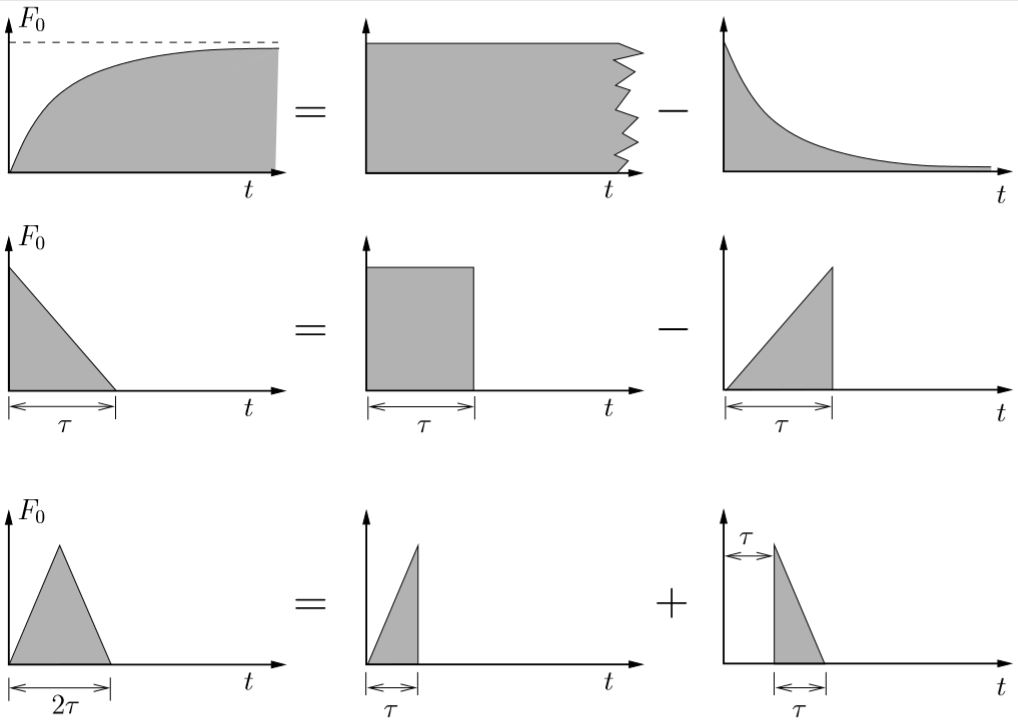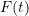### Transient Vibrations: Other Forcing Functions

So far we have considered the following forcing functions:

• harmonic,
• other periodic functions,
• ramp functions,
• exponential decay.

There are obviously many more we can come up with. Depending on the particular form of the forcing function, there are a number of ways of obtaining the response.

###### Simple Forcing Functions

For other simple forcing functions, they can sometimes be represented as combinations of the types we have already seen.Figure 7.7: Examples of composite forcing functions

In following this approach, we can sometime use superposition directly (as in the first two examples) or use two or more solutions sequentially where the final conditions for the first solution become the initial conditions for the second response.

###### Laplace Transforms

This approach transforms the differential equation into an algebraic equation which is significantly easier to solve in general. Once the solution is obtained, an inverse transform is performed to obtain the actual solution to the physical problem. This approach requires that the Laplace transform pairs are known for the particular forcing function of interest. We will not consider the use of Laplace transforms in this course, although they are very useful for vibration analysis and commonly used.

###### Convolution

This approach can be used for a general forcing functionand is based upon the response to impulsive loads that we have already discussed in equation (7.4). The method of convolution will be considered in the next section.